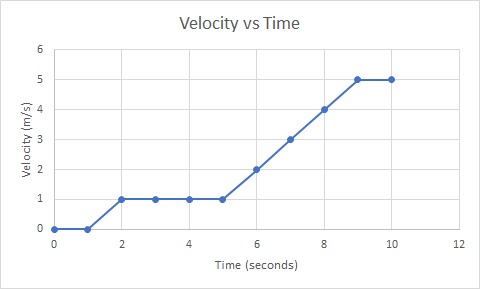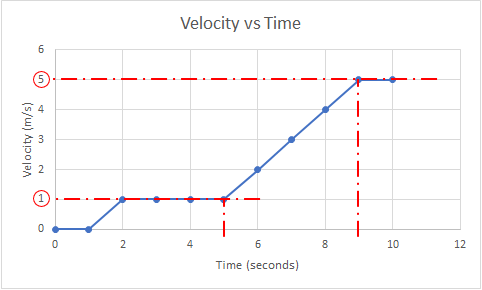## Velocity Plots

An electron's motion is tracked and plotted in the shown diagram. What is the electron's acceleration between the 5 and 9 second data points in m/s^2?Hint
Acceleration is defined as:
$$a=\frac{dv}{dt}$$$where $$v$$ is velocity and $$t$$ is time. Hint 2 $$a=\frac{dv}{dt}=\frac{\Delta v}{\Delta t}=\frac{v_{final}-v_{initial}}{t_{final}-t_{initial}}$$$
Acceleration is defined as:
$$a=\frac{dv}{dt}=\frac{\Delta v}{\Delta t}=\frac{v_{final}-v_{initial}}{t_{final}-t_{initial}}$$$where $$v$$ is velocity and $$t$$ is time. The plot’s x-axis is time and y-axis is velocity. Looking at the graph to find the corresponding velocity values associated with 5 and 9 second observation points:Therefore, $$v_{final}=5\:m/s$$ , $$v_{initial}=1\:m/s$$ , $$t_{final}=9\:s$$ , and $$t_{initial}=5\:s$$ . Plugging these values into the acceleration equation: $$a=\frac{5m/s-1m/s}{9s-5s}=\frac{4m/s}{4s}=1\:m/s^2$$$
$$1\:m/s^2$$\$